## Example Questions

### Example Question #1 : How To Find The Ratio Of A Fraction

Half of a salad is lettuce. A third of it is tomatoes. The remainder is made of cucumbers. Which of the following is the ratio of lettuce to cucumbers in the salad?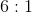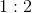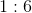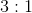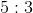Explanation:

First, you need to compute the amount of cucumbers used for the salad. A whole salad can be represented as(like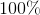). Thus, you know: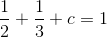First, simplify the part of the equation to the left of the equals sign: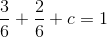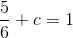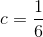Thus, the ratio of lettuce to cucumbers is: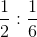This can be simplified by multiplying both sides by:### Example Question #2 : How To Find The Ratio Of A Fraction

One third of a diet bar is made of shredded fiber. Of the remaining portion, a third is made of apples and the remainder is made of soy. What is the ratio of shredded fiber to soy?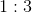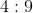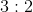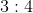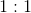Explanation:

We know that the bar could be represented as: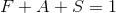, whererepresents. Now, we know that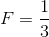. The remaining apples and soy comprise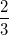of the bar. Now, the apples are one third of that remaining. (This is italicized because it is very important. The common error will be to think that it they are one third of the whole bar.) So, we know apples then are: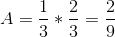Thus, we can compute the soy by subtracting that from the: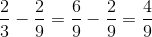Now the ratio of fiber to soy is: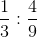Multiply both factors byto simplify:### Example Question #3 : How To Find The Ratio Of A Fraction

A pie is made up ofcrust,apples, andsugar, and the rest is jelly. What is the ratio of crust to jelly?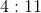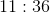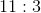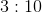Explanation:

A pie is made up ofcrust,apples,sugar, and the rest is jelly. What is the ratio of crust to jelly?

To compute this ratio, you must first ascertain how much of the pie is jelly. This is: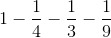Begin by using the common denominator: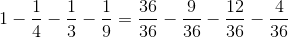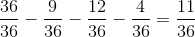So, the ratio of crust to jelly is: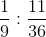This can be written as the fraction: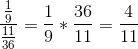, or### Example Question #4 : How To Find The Ratio Of A Fraction

In a solution,of the fluid is water,is wine, and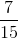is lemon juice. What is the ratio of lemon juice to water?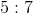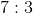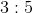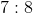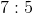Explanation:

This problem is really an easy fraction division. You should first divide the lemon juice amount by the water amount: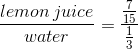Remember, to divide fractions, you multiply by the reciprocal: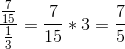This is the same as saying:### Example Question #5 : How To Find The Ratio Of A Fraction

If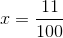and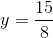, what is the ratio ofto?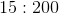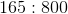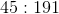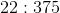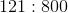Explanation:

To find a ratio like this, you simply need to make the fraction that represents the division of the two values by each other. Therefore, we have: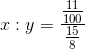Recall that division of fractions requires you to multiply by the reciprocal: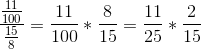which is the same as: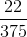This is the same as the ratio:### Example Question #1 : How To Find The Ratio Of A Fraction

In a certain school, for every three-eighths of a senior, there is one-half of a freshman. For every two freshmen, there are three-fourths of a junior. Which of the following could represent the ratio of juniors to seniors?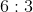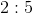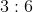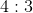Explanation:

Begin by manipulating the ratios into whole numbers. Let's begin with the ratio of seniors to freshmen. For every three-eights of a senior, there is one-half of a freshman: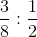In order to get rid of the denominators, multiply both sides by 8. Then our ratio becomes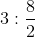or.

For every three seniors, there are four freshmen. Now let's do freshman to junior: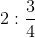Multiply both sides by four to get rid of the denominator. Our ratio now becomes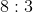.

For every 8 freshmen, there are 3 juniors. To find our final ratio, let's combine our previous two ratios by finding a common term (in this case freshmen). Because the ratio of seniors to freshmen is 3:4 and the ratio of juniors to freshmen is juniors is 8:3, multiply both sides of the first ratio to make the freshmen terms match.

Our new ratios now look like this:

6 seniors: 8 freshmen

8 freshmen: 3 juniors

Combine the two ratios using the common term:

6 seniors: 8 freshmen: 3 juniors

Take out the middle term:

6 seniors: 3 juniors

Therefore the ratio of seniors to juniors ismaking the ratio of juniors to seniors.

Tired of practice problems?

Try live online GRE prep today.